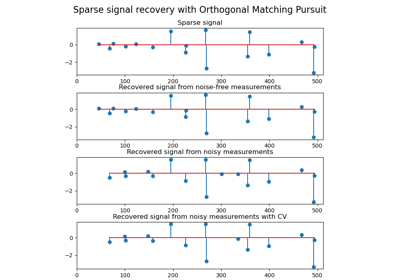# 3.2.4.1.8. sklearn.linear_model.OrthogonalMatchingPursuitCV¶

class sklearn.linear_model.OrthogonalMatchingPursuitCV(*, copy=True, fit_intercept=True, normalize=True, max_iter=None, cv=None, n_jobs=None, verbose=False)[source]

Cross-validated Orthogonal Matching Pursuit model (OMP).

See glossary entry for cross-validation estimator.

Read more in the User Guide.

Parameters
copybool, optional

Whether the design matrix X must be copied by the algorithm. A false value is only helpful if X is already Fortran-ordered, otherwise a copy is made anyway.

fit_interceptboolean, optional

whether to calculate the intercept for this model. If set to false, no intercept will be used in calculations (i.e. data is expected to be centered).

normalizeboolean, optional, default True

This parameter is ignored when fit_intercept is set to False. If True, the regressors X will be normalized before regression by subtracting the mean and dividing by the l2-norm. If you wish to standardize, please use sklearn.preprocessing.StandardScaler before calling fit on an estimator with normalize=False.

max_iterinteger, optional

Maximum numbers of iterations to perform, therefore maximum features to include. 10% of n_features but at least 5 if available.

cvint, cross-validation generator or an iterable, optional

Determines the cross-validation splitting strategy. Possible inputs for cv are:

• None, to use the default 5-fold cross-validation,

• integer, to specify the number of folds.

• An iterable yielding (train, test) splits as arrays of indices.

For integer/None inputs, KFold is used.

Refer User Guide for the various cross-validation strategies that can be used here.

Changed in version 0.22: cv default value if None changed from 3-fold to 5-fold.

n_jobsint or None, optional (default=None)

Number of CPUs to use during the cross validation. None means 1 unless in a joblib.parallel_backend context. -1 means using all processors. See Glossary for more details.

verboseboolean or integer, optional

Sets the verbosity amount

Attributes
intercept_float or array, shape (n_targets,)

Independent term in decision function.

coef_array, shape (n_features,) or (n_targets, n_features)

Parameter vector (w in the problem formulation).

n_nonzero_coefs_int

Estimated number of non-zero coefficients giving the best mean squared error over the cross-validation folds.

n_iter_int or array-like

Number of active features across every target for the model refit with the best hyperparameters got by cross-validating across all folds.

Examples

>>> from sklearn.linear_model import OrthogonalMatchingPursuitCV
>>> from sklearn.datasets import make_regression
>>> X, y = make_regression(n_features=100, n_informative=10,
...                        noise=4, random_state=0)
>>> reg = OrthogonalMatchingPursuitCV(cv=5).fit(X, y)
>>> reg.score(X, y)
0.9991...
>>> reg.n_nonzero_coefs_
10
>>> reg.predict(X[:1,])
array([-78.3854...])


Methods

 fit(X, y) Fit the model using X, y as training data. get_params([deep]) Get parameters for this estimator. Predict using the linear model. score(X, y[, sample_weight]) Return the coefficient of determination R^2 of the prediction. set_params(**params) Set the parameters of this estimator.
__init__(*, copy=True, fit_intercept=True, normalize=True, max_iter=None, cv=None, n_jobs=None, verbose=False)[source]

Initialize self. See help(type(self)) for accurate signature.

fit(X, y)[source]

Fit the model using X, y as training data.

Parameters
Xarray-like, shape [n_samples, n_features]

Training data.

yarray-like, shape [n_samples]

Target values. Will be cast to X’s dtype if necessary

Returns
selfobject

returns an instance of self.

get_params(deep=True)[source]

Get parameters for this estimator.

Parameters
deepbool, default=True

If True, will return the parameters for this estimator and contained subobjects that are estimators.

Returns
paramsmapping of string to any

Parameter names mapped to their values.

predict(X)[source]

Predict using the linear model.

Parameters
Xarray_like or sparse matrix, shape (n_samples, n_features)

Samples.

Returns
Carray, shape (n_samples,)

Returns predicted values.

score(X, y, sample_weight=None)[source]

Return the coefficient of determination R^2 of the prediction.

The coefficient R^2 is defined as (1 - u/v), where u is the residual sum of squares ((y_true - y_pred) ** 2).sum() and v is the total sum of squares ((y_true - y_true.mean()) ** 2).sum(). The best possible score is 1.0 and it can be negative (because the model can be arbitrarily worse). A constant model that always predicts the expected value of y, disregarding the input features, would get a R^2 score of 0.0.

Parameters
Xarray-like of shape (n_samples, n_features)

Test samples. For some estimators this may be a precomputed kernel matrix or a list of generic objects instead, shape = (n_samples, n_samples_fitted), where n_samples_fitted is the number of samples used in the fitting for the estimator.

yarray-like of shape (n_samples,) or (n_samples, n_outputs)

True values for X.

sample_weightarray-like of shape (n_samples,), default=None

Sample weights.

Returns
scorefloat

R^2 of self.predict(X) wrt. y.

Notes

The R2 score used when calling score on a regressor uses multioutput='uniform_average' from version 0.23 to keep consistent with default value of r2_score. This influences the score method of all the multioutput regressors (except for MultiOutputRegressor).

set_params(**params)[source]

Set the parameters of this estimator.

The method works on simple estimators as well as on nested objects (such as pipelines). The latter have parameters of the form <component>__<parameter> so that it’s possible to update each component of a nested object.

Parameters
**paramsdict

Estimator parameters.

Returns
selfobject

Estimator instance.

## 3.2.4.1.8.1. Examples using sklearn.linear_model.OrthogonalMatchingPursuitCV¶